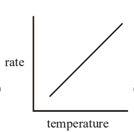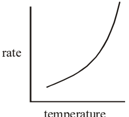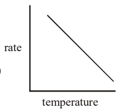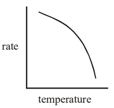The following data are obtained from the decomposition of a gaseous compound

Initial pressure, atm               1.6       0.8       0.4

Time for 50% reaction, min     80       113      160

The order of the reaction is

(A) 1.0

(B) 1.5

(C) 2.0

(D) 0.5

High Yielding Test Series + Question Bank - NEET 2020

Difficulty Level:

For a given reaction the concentration of the reactant plotted against time gave a straight line with negative slope.

The order of the reaction is-

(A) 3

(B) 2

(C) 1

(D) 0

High Yielding Test Series + Question Bank - NEET 2020

Difficulty Level:

The rate constant of a first order reaction is generally determined from a plot of

(A) Concentration of reactant vs time t

(B) log (concentration of reactant) vs time t

(C)

(D) Concentration of reactant vs log time t

High Yielding Test Series + Question Bank - NEET 2020

Difficulty Level:

Which of the following correctly represents the variation of the rate of the reaction with temperature ?

(A)(B)(C)(D)High Yielding Test Series + Question Bank - NEET 2020

Difficulty Level:

The plot of log k versus $\frac{1}{T}$is linear with a slope of

(A) $\frac{{E}_{a}}{R}$

(B) $\frac{-{E}_{a}}{R}$

(C) $\frac{{E}_{a}}{2.303R}$

(D) $\frac{-{E}_{a}}{2.303R}$

High Yielding Test Series + Question Bank - NEET 2020

Difficulty Level:

If a reaction A + B$\to$ C is exothermic to the extent of 30 kJ/mol and the forward reaction has an activation energy 70 kJ/mol, the activation energy for the reverse reaction is

(A) 30 kJ/mol

(B) 40kJ/mol

(C) 70 kJ/mol

(D) 100 kJ/mol

High Yielding Test Series + Question Bank - NEET 2020

Difficulty Level:

A first order reaction takes 40 min for 30% decomposition.Calculate ${t}_{1/2}$

(A) 77.7 min.

(B) 27.2 min.

(C) 55.3 min.

(D) 67.3 min.

Concept Questions :-

First Order Reaction Kinetics
High Yielding Test Series + Question Bank - NEET 2020

Difficulty Level:

For a certain reaction involving a single reactant, it is found that ${C}_{0}\sqrt{T}$ is constant where ${C}_{0}$is the initial concentration of the reactant and T is the half-life. What is the order of the reaction ?

(A) 1

(B) Zero

(C) 2

(D) 3

Concept Questions :-

Order and Molecularity
High Yielding Test Series + Question Bank - NEET 2020

Difficulty Level:

The high temperature ($\approx$ 1200K) decomposition of $C{H}_{3}COOH$(g) occurs as follows as per simultaneous 1st order reactions.

What would be the % of $C{H}_{4}$by mole in the product mixture (excluding$C{H}_{3}COOH$) ?

(A)

(B)

(C)

(D) it depends on time

Concept Questions :-

First Order Reaction Kinetics
High Yielding Test Series + Question Bank - NEET 2020

Difficulty Level:

The reaction , A(g) + 2B(g) C(g) + D(g) is an elementary process. In an experiment, the initial partial pressure of A and B are ${P}_{A}$= 0.60 and ${P}_{B}$= 0.80 atm. When ${P}_{C}$= 0.2atm the rate of reaction relative to the initial rate is

(A) 1/48

(B) 1/24

(C) 9/16

(D) 1/6

Concept Questions :-

First Order Reaction Kinetics